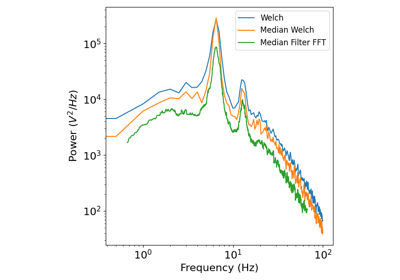# neurodsp.spectral.rotate_powerlaw¶

neurodsp.spectral.rotate_powerlaw(freqs, spectrum, delta_exponent, f_rotation=1)[source]

Rotate the power law exponent of a power spectrum.

Parameters
freqs1d array

Frequency axis of input spectrum, in Hz.

spectrum1d array

Power spectrum to be rotated.

delta_exponentfloat

Change in power law exponent to be applied. Positive is clockwise rotation (steepen), negative is counter clockwise rotation (flatten).

f_rotationfloat, optional, default: 1

Frequency, in Hz, to rotate the spectrum around, where power is unchanged by the rotation. This only matters if not further normalizing signal variance.

Returns
rotated_spectrum1d array

Rotated spectrum.

Examples

Rotate a power spectrum, calculated on simulated data:

>>> from neurodsp.sim import sim_combined
>>> from neurodsp.spectral import compute_spectrum
>>> sig = sim_combined(n_seconds=10, fs=500,
...                    components={'sim_powerlaw': {}, 'sim_oscillation' : {'freq': 10}})
>>> freqs, spectrum = compute_spectrum(sig, fs=500)
>>> rotated_spectrum = rotate_powerlaw(freqs, spectrum, -1)


## Examples using neurodsp.spectral.rotate_powerlaw¶